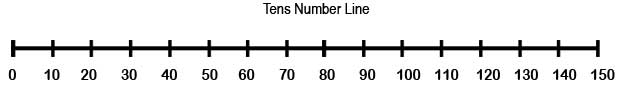Rounding: Tens Rounding numbers makes numbers easier to work with in your head. Round numbers will end with 0. It is an estimate or "about this much" - "About 50 students came to the game." Even though there were exactly 53. The column below (to the right of) the place value to round to will tell which number to round to. 1,2,3 & 4: Round down. 5,6,7,8,9: Round up.
 Directions: Round numbers to the nearest 10. Write the ten below & the ten above the given number. Circle which ten you round to. Example: Round to the nearest ten: 84 - The number line shows 80 as the ten below 84 and 90 as the ten above. Or the place value next the tens is the ones. (84) 4 is in the ones & 4 says Round Down to 80. Name _____________________________ Date: ______________________________ Score: __________________________1.
30

 26

20
2.
70

 63

60
3.
100

 95

90
4.

 14

5.

 63

6.

 87

7.

 91

8.

 31

9.

 46

10.

 54

11.

 69

12.

 82

13.

 15

14.

 21

15.

 19

16.

 58

17.

 43

18.

 28

19.

 8

20.

 89

21.

 72

22.

 66

23.

 34

24.

 43

25.

 18